# Changing Outcomes in Blockly - Part One (Ages 9-13)

Hello everyone! Today we are going to practice changing the outcome of a code in Blockly. By the end of the activity, you’ll be able to understand what an outcome is, and how to change it by changing the order of the blocks!

Before we begin:

1. Make sure that you have your KINI robot, and that it is connected to the internet.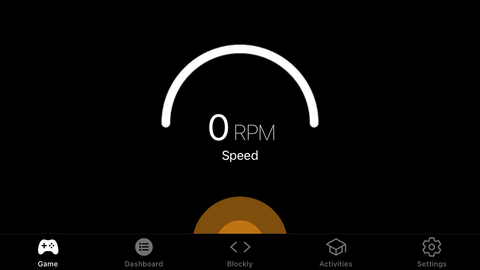2. Open the app, and go to the Blockly tab by clicking the Blockly button in the bottom right corner. We will be using the two Repeat blocks to create our code.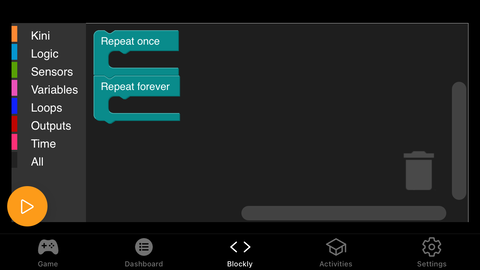3. Understand the basics of Blockly - if you don’t, go to the “Introduction to Blockly” activity before this one.

What is an outcome?

• In coding, an outcome is the result of a code. In our case, the outcome is KINI’s movement, or where KINI is after moving.
• In this activity we are going to start with an existing code, and learn how to change it, to make a new outcome.

Outcomes That Don’t Depend on the Order of Blocks

• An example of outcomes that don’t depend on the order of blocks, is a sequence with just move forward and backward blocks
• Below, you can see the use of a move forward for 20cm block and a move backwards for 40 cm in both orders in Blockly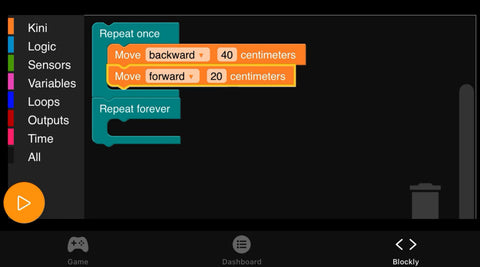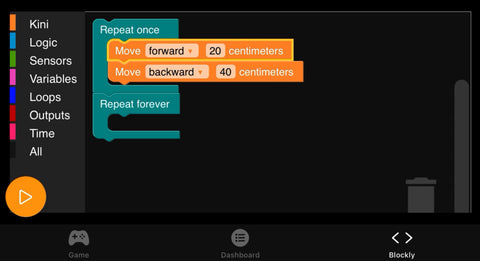• The outcome of this code (in both orders) is that KINI will end up 20cm backwards from where it started, as 40cm backwards minus 20 cm forwards is 20cm backwards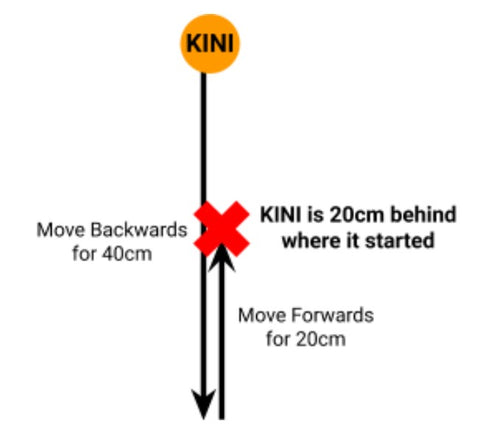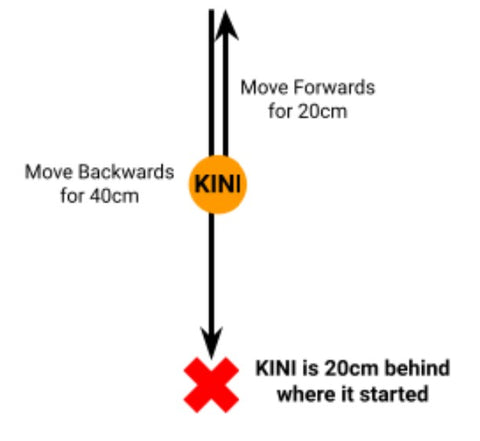• If you don’t understand, you can draw this example to see that the outcome is 20cm backwards

Outcomes That Depend on the Order of Blocks:

• An example of where the order matters, is if you add a rotate block to the sequence
• Below, you can see the results depending on where a Rotate Left block is placed in the sequence from the previous example
• If the rotate block is at the start of the sequence, KINI would end up to the right of where it started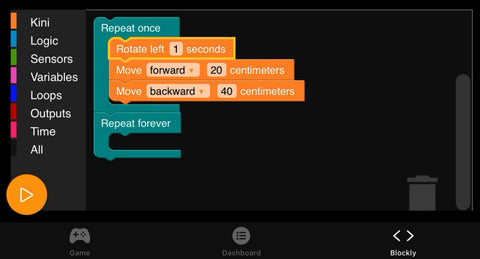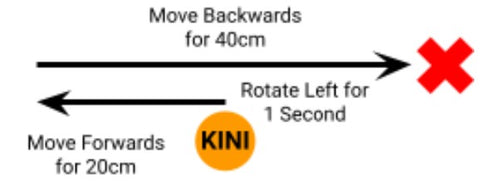• If the rotate block is at the end of the sequence, KINI would end up behind where it started• As you can see, the order of the blocks impacts KINI’s final position
• Using this code, you can also find out how KINI’s ending position will change if you change the order of the same three blocks

Congratulations everyone! You just learnt what an outcome is, and how to change it by changing the order of blocks. Since the outcome of a code can change by rearranging the same blocks, it is a good idea to draw KINI’s movements. That way, you can understand how the order impacts the outcome. You can try out this same code, and even try changing it by rearranging the blocks to get a new outcome.

Now that you’ve learnt about outcomes, each time you code KINI, first think of the outcome, then use blocks to make that outcome happen. Good luck, and have fun coding!